# Management Accounting Breakeven Analysis Breakeven Analysis Defined o

• Slides: 17Management Accounting Breakeven AnalysisBreakeven Analysis Defined o o Breakeven analysis examines the short run relationship between changes in volume and changes in total sales revenue, expenses and net profit Also known as C-V-P analysis (Cost Volume Profit Analysis)Uses of Breakeven Analysis o o o C-V-P analysis is an important tool in terms of short -term planning and decision making It looks at the relationship between costs, revenue, output levels and profit Short run decisions where C-V-P is used include choice of sales mix, pricing policy etc.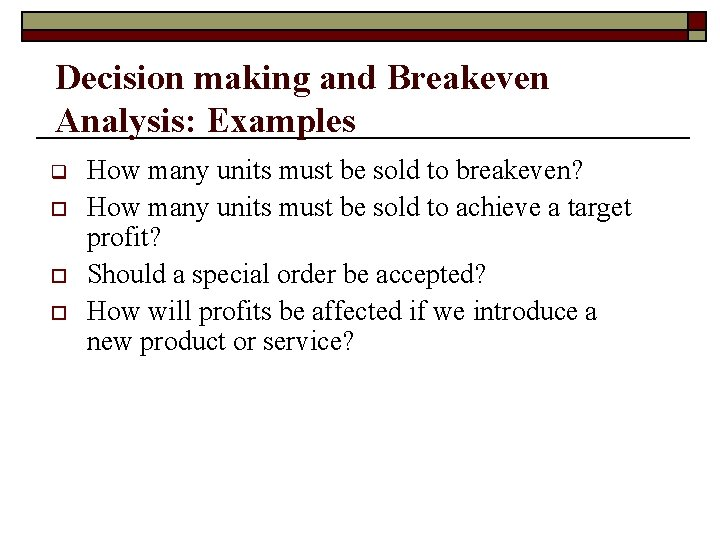Decision making and Breakeven Analysis: Examples q o o o How many units must be sold to breakeven? How many units must be sold to achieve a target profit? Should a special order be accepted? How will profits be affected if we introduce a new product or service?Key Terminology: Breakeven Analysis o o Break even point-the point at which a company makes neither a profit or a loss. Contribution per unit-the sales price minus the variable cost per unit. It measures the contribution made by each item of output to the fixed costs and profit of the organisation.Key Terminology ctd. o o Margin of safety-a measure in which the budgeted volume of sales is compared with the volume of sales required to break even Marginal Cost – cost of producing one extra unit of output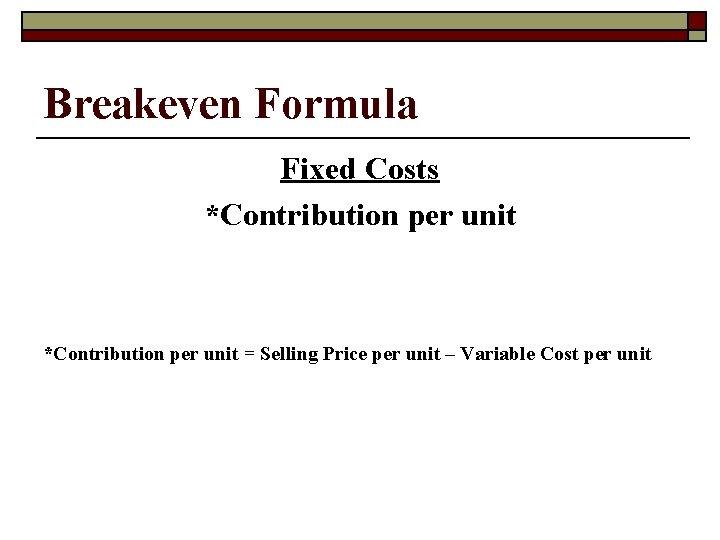Breakeven Formula Fixed Costs *Contribution per unit = Selling Price per unit – Variable Cost per unitBreakeven ChartMargin of Safety o o o The difference between budgeted or actual sales and the breakeven point The margin of safety may be expressed in units or revenue terms Shows the amount by which sales can drop before a loss will be incurred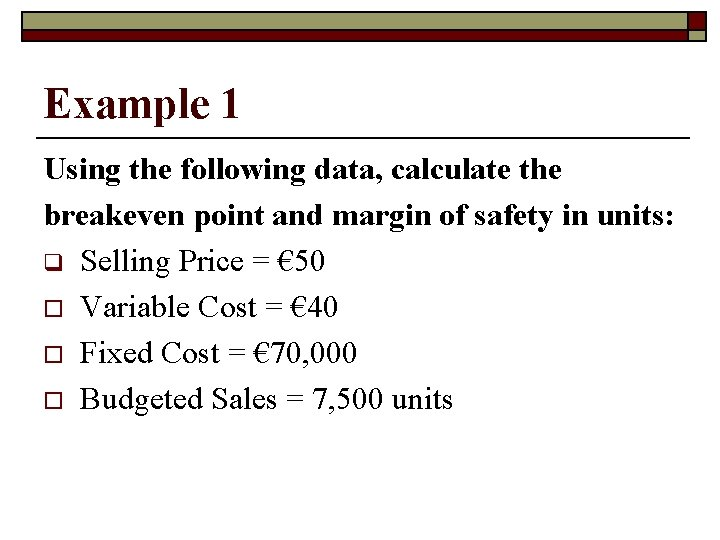Example 1 Using the following data, calculate the breakeven point and margin of safety in units: q Selling Price = € 50 o Variable Cost = € 40 o Fixed Cost = € 70, 000 o Budgeted Sales = 7, 500 units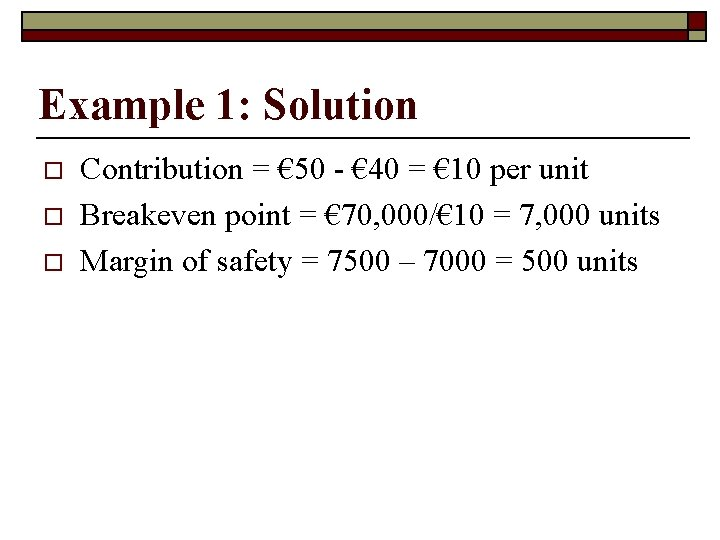Example 1: Solution o o o Contribution = € 50 - € 40 = € 10 per unit Breakeven point = € 70, 000/€ 10 = 7, 000 units Margin of safety = 7500 – 7000 = 500 units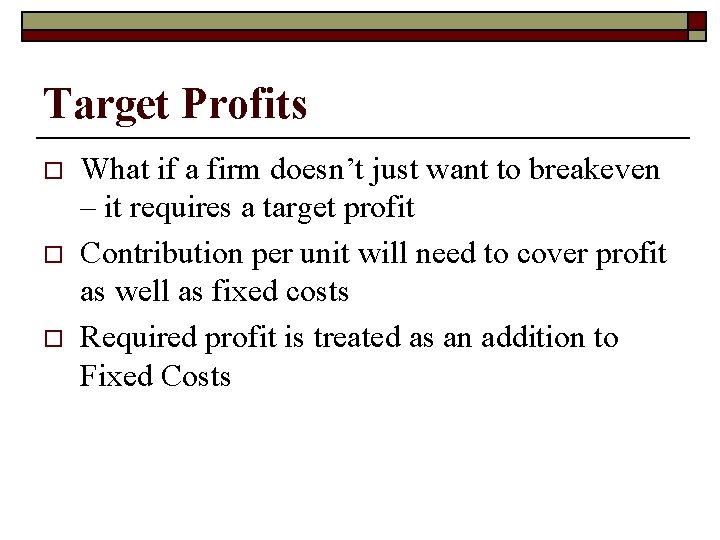Target Profits o o o What if a firm doesn’t just want to breakeven – it requires a target profit Contribution per unit will need to cover profit as well as fixed costs Required profit is treated as an addition to Fixed Costs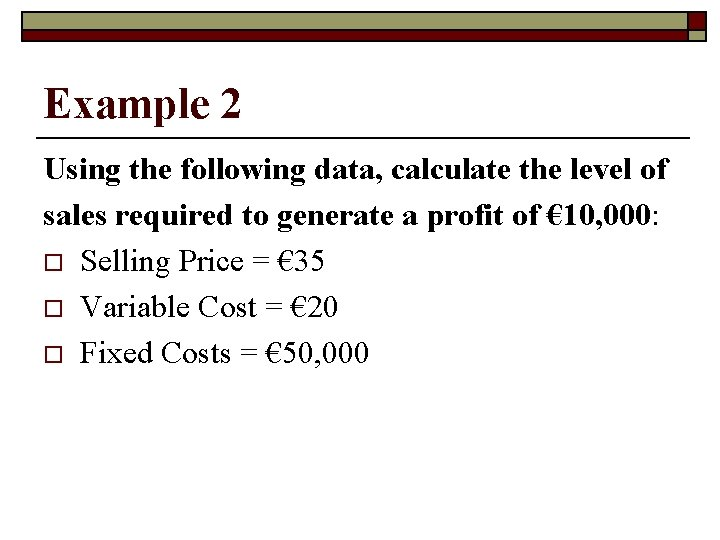Example 2 Using the following data, calculate the level of sales required to generate a profit of € 10, 000: o Selling Price = € 35 o Variable Cost = € 20 o Fixed Costs = € 50, 000Example 2: Solution o o Contribution = € 35 – € 20 = € 15 Level of sales required to generate profit of € 10, 000: € 50, 000 + € 10, 000 € 15 4000 units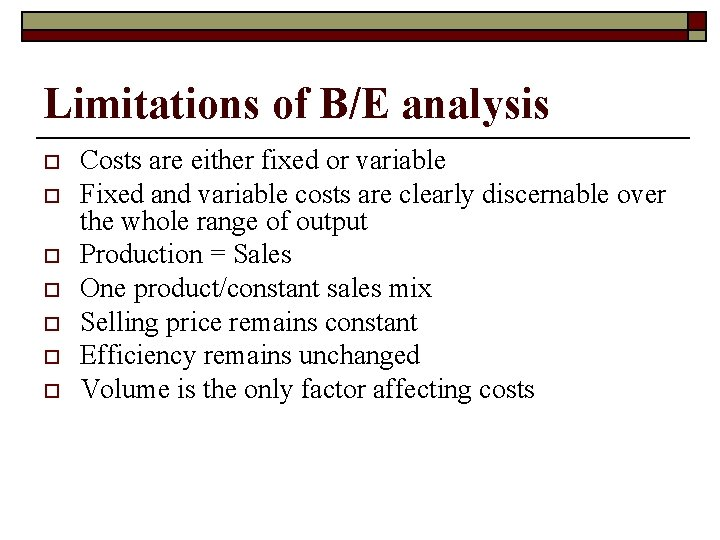Limitations of B/E analysis o o o o Costs are either fixed or variable Fixed and variable costs are clearly discernable over the whole range of output Production = Sales One product/constant sales mix Selling price remains constant Efficiency remains unchanged Volume is the only factor affecting costs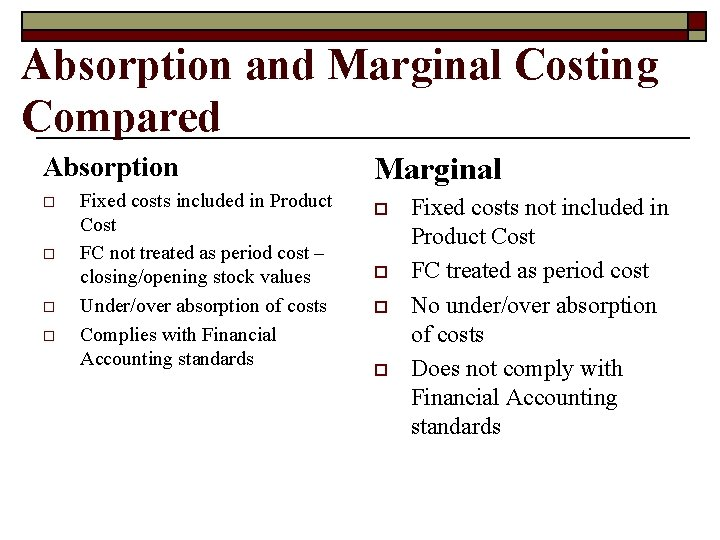Absorption and Marginal Costing Compared Absorption o o Fixed costs included in Product Cost FC not treated as period cost – closing/opening stock values Under/over absorption of costs Complies with Financial Accounting standards Marginal o o Fixed costs not included in Product Cost FC treated as period cost No under/over absorption of costs Does not comply with Financial Accounting standards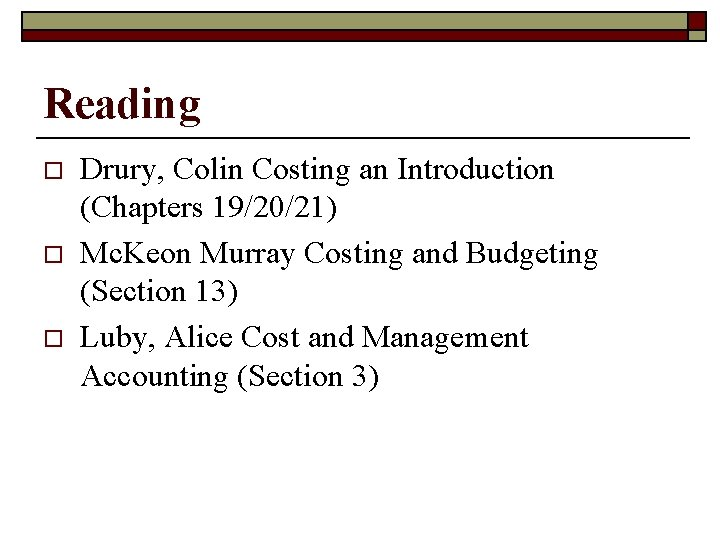Reading o o o Drury, Colin Costing an Introduction (Chapters 19/20/21) Mc. Keon Murray Costing and Budgeting (Section 13) Luby, Alice Cost and Management Accounting (Section 3)